# Resistances in Series and Resistances in Parallel

More than one electrical resistance can be connected either in series or in parallel in addition to that, more than two resistances can also be connected in combination of series and parallel both. Here we will discuss mainly about series and parallel combination.

## Resistances in Series

Suppose you have three different types of resistors – R1, R2 and R3 – and you connect them end to end as shown in the figure below, then it would be referred as resistances in series. In case of series connection, the equivalent resistance of the combination, is sum of these three electrical resistances.
That means, resistance between point A and D in the figure below, is equal to the sum of three individual resistances. The current enters in to the point A of the combination, will also leave from point D as there is no other parallel path provided in the circuit.

Now say this current is I. So this current I will pass through the resistance R1, R2 and R3. Applying Ohm’s law, it can be found that voltage drops across the resistances will be V1 = IR1, V2 = IR2 and V3 = IR3. Now, if total voltage applied across the combination of resistances in series, is V.
Then obviously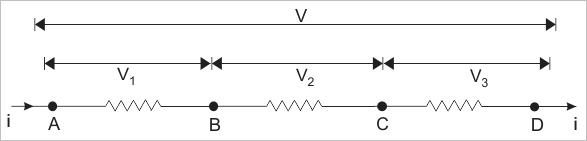Since, sum of voltage drops across the individual resistance is nothing but the equal to applied voltage across the combination.

Now, if we consider the total combination of resistances as a single resistor of electric resistance value R, then according to Ohm’s law,
V = IR ………….(2)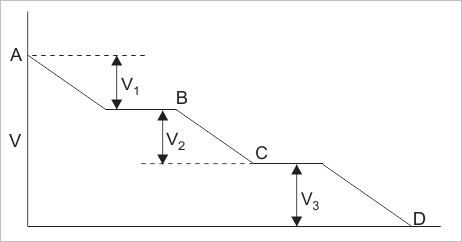Now, comparing equation (1) and (2), we get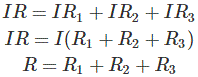So, the above proof shows that equivalent resistance of a combination of resistances in series is equal to the sum of individual resistance. If there were n number of resistances instead of three resistances, the equivalent resistance will be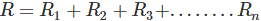## Resistances in Parallel

Say we have three resistors of resistance value R1, R2 and R3. These resistors are connected in such a manner that the right and left side terminal of each resistor is connected together, as shown in the figure below.This combination is called resistances in parallel. If electric potential difference is applied across this combination, then it will draw a current I (say).
As this current will get three parallel paths through these three electrical resistances, the current will be divided into three parts. Say currents I1, I1 and I1 pass through resistor R1, R2 and R3 respectively.
Where total source current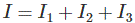Now, as from the figure it is clear that, each of the resistances in parallel, is connected across the same voltage source, the voltage drops across each resistor is the same, and it is same as supply voltage V (say).
Hence, according to Ohm’s law,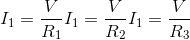Now, if we consider the equivalent resistance of the combination is R.
Then,Now putting the values of I, I1, I2 and I3 in equation (1) we get,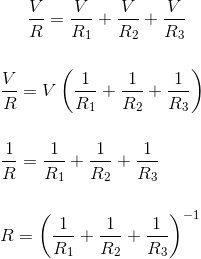The above expression represents equivalent resistance of resistor in parallel. If there were n number of resistances connected in parallel, instead of three resistances, the expression of equivalent resistance would be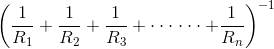Want To Learn Faster? 🎓
Get electrical articles delivered to your inbox every week.
No credit card required—it’s 100% free.